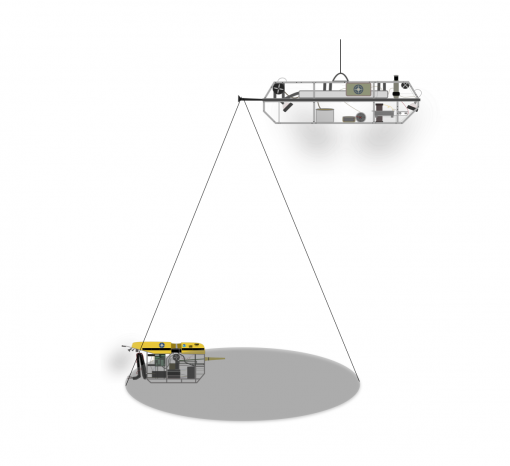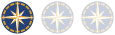Education

# Tether Trigonometry

STEM Learning ModuleOcean Exploration Trust

Gain a practical application for right triangle trigonometry (Pythagorean theorem) by solving for vertical and linear distance relationships between remotely operated vehicles Hercules and Argus during dive operations. Building on these skills, students can calculate the operational area (area of a circle) based on different vehicle configurations. Worksheet problems challenge students to use multiple equation strategies to solve these underwater problems.

## Objectives & learning outcomes

Students will:

• determine unknown side lengths in right triangles.
• calculate the area of a circle by solving for the radius, using real-world problems and trigonometry.

## Time & materials

Time & materialsPrep time and materials cost minimal, may require printing.

Documents